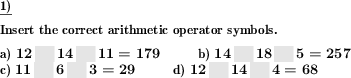Custom math worksheets at your fingertips# Details for problem "Insert two arithmetic operators"

Quickname: 6624

Elementary School, Primary School, Junior High School, Middle School, High School.

## Summary

In an equation with three operands, insert two operators.

## Example## Description

An equation with three operands and two free spaces for arithmetic operators is given. Possible operations are:

• Subtraction
• Multiplication
• Division

The correct arithmetic operator symbols have to be chosen and put in the blank space so that the equation is correct.

The number of problems and the range of the operators can be chosen.

For the operators, these types of combinations can be selected:

• one operator in multiply, the other is add or subtract
• one operator is add or subtract, the other is multiply or divide
• both operators can be one of add, subtract or multiply
• both operators can be one of add, subtract, multiply and divide

Download free printable worksheets for this math problem here. The worksheet contains the problems only, the solution sheet includes the answers. Just click on the respective link.

•Worksheet 1Solution sheet with answers
•Worksheet 2Solution sheet with answers
•Worksheet 3Solution sheet with answers

If you can not see the solution sheets for download, they may be filtered out by an ad blocker that you may have installed. If this is the case, please allow ads for this page and reload the page. The solution sheets will then reappear.

• Do these sample worksheets do not really fit?
• Do you need more math worksheets, with a different level of difficulty?
• Would you like to combine different problems on a worksheet and adjust them to your needs?
• As a teacher, you can put together your own worksheets using the automatically generated math problems provided.
With a free initial credit, you can start creating your own math worksheets in a few minutes.

You can try it for free! Register here, to create custom worksheets now!

## Customization options for this problem

Parameter
Possible values
Number of problems
1, 2, 3, 4, 5, 6, 7, 8, 9, 10
Operands number range
10, 20, 30, 40, 50, 100, 200, 500, 1000, 10000, 100000
Operators

## Similar problems

Remark
Description
With only one operator and two operands
In an equation with two operands, insert the correct arithmetic symbol.